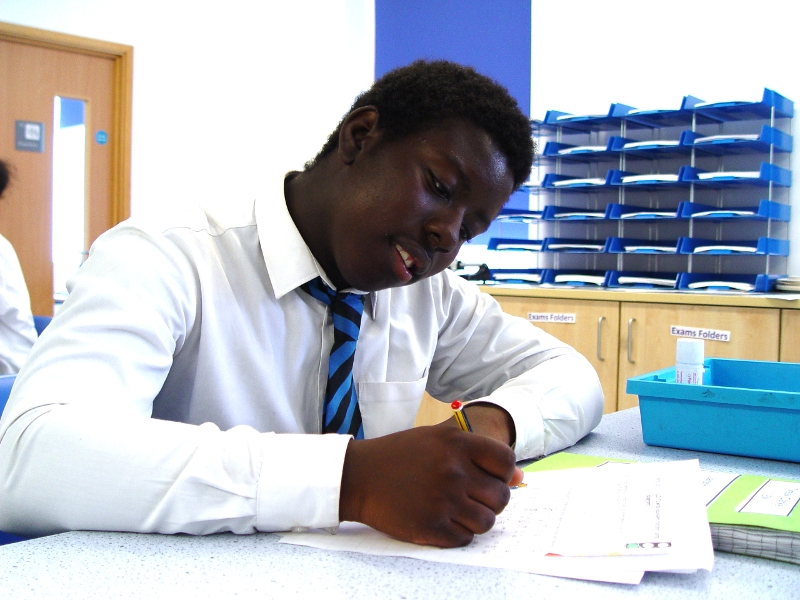# Year 11 End of Term Maths LearningIt may be coming towards the end of term but Year 11 have been continuing to work hard on their maths knowledge. We usually start each lesson with a times table challenge. The students spend five minutes on answering various multiplication questions.

Each student has a unique learning target. Teaching staff prepare challenges that help the class members to reach their goals.

One student has been writing equivalent fractions using diagrams. Another activity has involved solving multi-step word problems using addition and subtraction. The trick here is to read the questions carefully and work out what sums are needed to get to the final answer.

A final learning intention was to solve the magical decimal squares. This involved making sure that rows, columns and diagonals in a grid had a decimal that made the magical decimal sum correct in the centre.